Courses

# Electromagnetic Induction MCQ Level - 2

## 10 Questions MCQ Test Topic wise Tests for IIT JAM Physics | Electromagnetic Induction MCQ Level - 2

Description
This mock test of Electromagnetic Induction MCQ Level - 2 for Physics helps you for every Physics entrance exam. This contains 10 Multiple Choice Questions for Physics Electromagnetic Induction MCQ Level - 2 (mcq) to study with solutions a complete question bank. The solved questions answers in this Electromagnetic Induction MCQ Level - 2 quiz give you a good mix of easy questions and tough questions. Physics students definitely take this Electromagnetic Induction MCQ Level - 2 exercise for a better result in the exam. You can find other Electromagnetic Induction MCQ Level - 2 extra questions, long questions & short questions for Physics on EduRev as well by searching above.
QUESTION: 1

### An inductor L and a resistor R are connected in series with a direct current source of emf E. The maximum rate at which energy is stored in the magnitude field is :

Solution:

The graph of current is given by :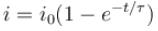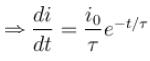Energy stored in the form of magnetic field energy is :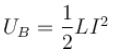Rate of increase of magnetic field energy is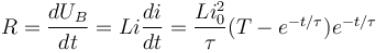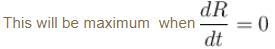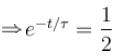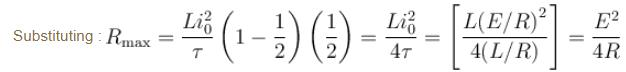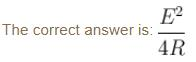QUESTION: 2

### In the figure shown a square loop PQRS  of side ‘a’  and resistance ‘r ’  is placed near an infinitely long wire carrying a constant current I. The sides PQ and  RS  are parallel to the wire. The wire and the loop are in the same plane. The loop is rotated by 180° about an axis parallel to the long wire and passing through the mid points of the side QR  and  PS. The total amount of charge which passes through any point of the loop during rotation is :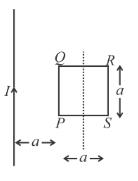Solution: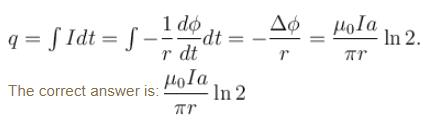QUESTION: 3

### PQ  is an infinite current carrying conductor. AB and CD are smooth conducting rods on which a conductor EF  moves with constant velocity V as shown. The force needed to maintain constant speed of EF  is :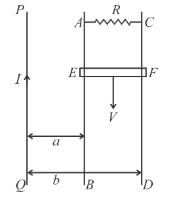Solution: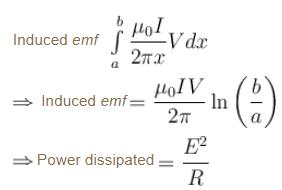Also, power = F.V.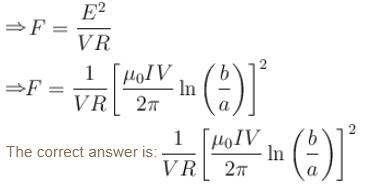QUESTION: 4

A superconducting loop of radius R has self inductance L. A uniform and constant magnetic field B is applied perpendicular to the plane of the loop. Initially current in this loop is zero. The loop is rotated by 180°. The current in the loop after rotation is equal to :

Solution:

Flux can’t change in a superconducting loop.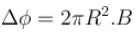Initially current was zero. So self flux was zero.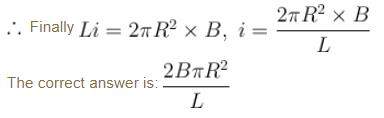QUESTION: 5

A wooden stick of length 3l  is rotated about an end with constant angular velocity ω in a uniform magnetic field B perpendicular to the plane of motion. If the upper one third of its length is coated with copper, the potential across the whole length of the stick is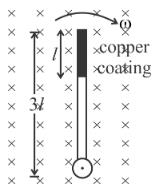Solution:

When the rod rotates, there will be an induced current in the rod. The given situation can be treated as if a rod A  of length ‘3l rotating in the clockwise direction, while an other say rod B of length ‘2l rotating in the anti clockwise direction with same angular speed ω.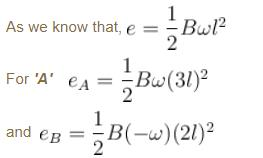Resultant induced emf  will be :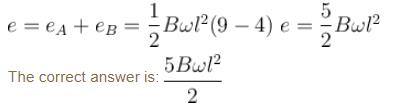QUESTION: 6

A uniform magnetic field of induction B is confined to a cylindrical region of radius R. The magnetic field is increasing at a constant rate of dB/dt (tesla/second). An electron of charge q, placed at the point P on the periphery of the field experiences an acceleration :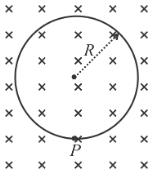Solution:

If we consider the cylindrical surface to be a ring of radius  R, there will be an induced emf due to changing field.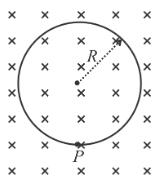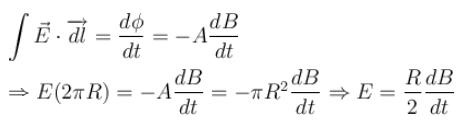∴  Force on the electron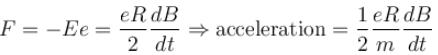As the field is increasing being directed inside the paper, hence there will be anticlockwise induced current (in order to oppose the cause) in the ring (assumed). Hence there will be a force towards left on the electron.

The correct answer is: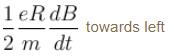QUESTION: 7

A rod of length l having uniformly distributed charge Q is rotated about one end with constant frequency f ’. Its magnetic moment is :

Solution:

Charge on the differential element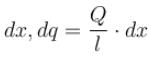equivalent current  di = f dq
∴  magnetic moment of this element, (N = 1)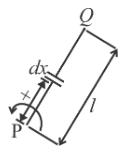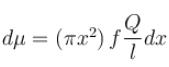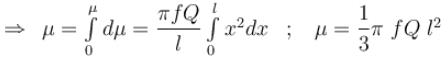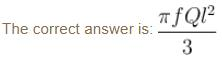QUESTION: 8

A metallic ring of mass m  and radius r with a uniform metallic spoke of same mass m and length r is rotated about its axis with angular velocity ω in a perpendicular uniform magnetic field B as shown. If the central end of the spoke is connected to the rim of the wheel through a resistor R as shown. The resistor does not rotate, its one end is always at the center of the ring and other end is always in contact with the ring. A force F as shown is needed to maintain constant angular velocity of the wheel. F is equal to (The ring and the spoke has zero resistance)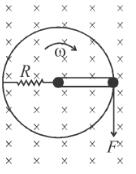Solution:

Fb = BIL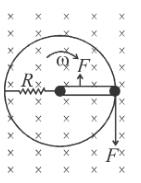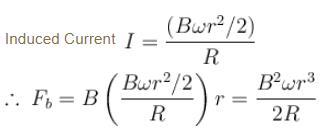To maintain constant angular velocity :
F(r) = FB(r/2)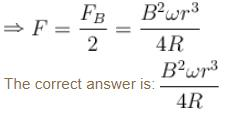QUESTION: 9

In the circuit shown in figure, the switch S was initially at position 1. After sufficiently long time, the switch S was thrown from position 1 to position 2. The voltage drop across the resistor at that instant is :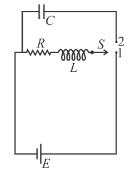Solution:

When the switch is at position 1 :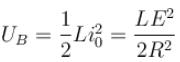Just after the switch is shifted to position 2, current I = E/R is flowing across the resistance. Hence at that instant potential difference across resistance will be :

QUESTION: 10

A rectangular loop of sides a and  b is placed in xy plane. A very long wire is also placed in xy plane such that side of length a of the loop is parallel to the wire. The distance between the wire and the nearest edge of the loop is d ’. The mutual inductance of this system is proportional to :

Solution:

The flux in rectangular loop due to current in wire is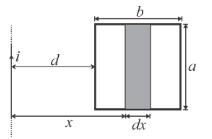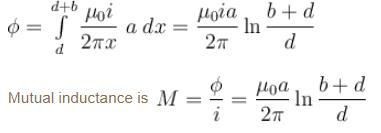∴  Mutual inductance is proportion to  'a'.